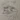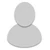## FANDOM

2,287 Pages

• option A def

• Option B, I guess?

• EeveeWonders wrote: Option B, I guess?

•Biesaga removed this reply because: fixeed :))))))))))))))))))))))))))) 00:44, February 29, 2016
• Biesaga wrote: Nooooo why was option b deleted

wha

• Option A

• Notorious Onion wrote:

Biesaga wrote: Nooooo why was option b deleted

wha

it was deleted but then it was put back B)))))))))))))))))))))))))

• Notorious Onion wrote:

EeveeWonders wrote: Option B, I guess?

• Biesaga wrote:
Notorious Onion wrote:

EeveeWonders wrote: Option B, I guess?

• Lol, why is this a thing?

• Live Delta wrote:
Lol, why is this a thing?

Because it's very important.

•